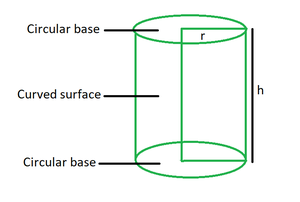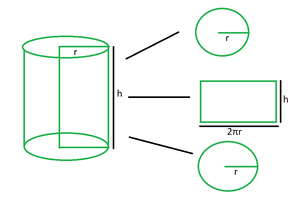# Surface Area of a Cylinder Formula

• Last Updated : 31 Mar, 2022

Mensuration is a discipline of mathematics that deals with measurements by calculating the height, breadth, depth, perimeter, area, and volume of a single item or set of objects. It describes numerous geometrical shapes, such as two-dimensional shapes, as well as their attributes and the formulae that are utilised. It applies to two-dimensional forms like squares, rectangles, and triangles, as well as three-dimensional objects like cubes, cuboids, and cylinders.

### Cylinder

A cylinder is a three-dimensional structure formed by two parallel circular bases connected by a curving surface. The circular bases’ centres overlap each other to form a right cylinder. The axis is the line segment that connects the two centres and represents the height of the cylinder. The top view of the cylinder resembles a circle, while the side view resembles a rectangle. A cylinder, unlike a cone, cube, or cuboid, lacks vertices due to its curved form and lack of straight lines. It features two concentric circles on its face.### Surface Area of Cylinder

A cylinder’s surface area is defined as the amount of space covered by the flat surface of the cylinder’s bases and the curved surface of the cylinder. The overall surface area of the cylinder includes the area of the cylinder’s two circular bases as well as the area of the curving surface. The cylinder’s surface area formula is used to calculate the surface area occupied by the cylinder’s bases and curving surface. Because a cylinder has a curved surface, we may express both its curved surface area and overall surface area. A cylinder has two types of surface areas: total surface area and curved surface area. We will discuss both of them one by one.

Curved Surface Area of a cylinder

The curved surface area of the cylinder is enclosed between the two parallel circular bases. It is also known as the lateral surface area. The formula is as follows:

Curved surface area = 2πrh

where,

r = radius of the cylinder

h = height of cylinder

Total Surface Area of a cylinder

A cylinder’s total surface area is the sum of its curved surface area and the area of its two circular bases. It is calculated by summing the areas of the two bases and the curved surface. As a result, the formula for the cylinder’s total surface area is as follows:

Total cylinder surface area = 2πr2 + 2πrh = 2πr(r + h)

where,

r = radius of the cylinder

h = height of cylinder

Derivation

Consider a cylinder whose radius is r and height is h.The cylinder is divided into three parts: one circular base, one rectangular area and another circular base.

The rectangular area has length of 2πr and breadth of h. So, the area is, A1 = 2πrh, which is also the curved surface area of the cylinder.

The area of a circular base with radius r is given by, πr2. So, the area of two such bases is, A2 = (πr2 + πr2) = 2πr2.

Now, the total surface area of the cylinder is the sum of above two areas.

A = A1 + A2

= 2πr2 + 2πrh

= 2πr(r + h)

This derives the formula for surface area of a cylinder.

### Sample Questions

Question 1. Find the curved surface area of the cylinder of radius 3 cm and height of 7 cm.

Solution:

We have, r = 3 and h = 7.

Curved surface area of cylinder = 2πrh

= 2 (22/7) (3) (7)

= 2 (22) (3)

= 132 cm2

Question 2. Find the curved surface area of the cylinder of radius 5 cm and height of 14 cm.

Solution:

We have, r = 5 and h = 14.

Curved surface area of cylinder = 2πrh

= 2 (22/7) (5) (14)

= 2 (22) (5) (2)

= 440 cm2

Question 3. Find the radius of the cylinder of curved surface area 220 sq. cm and height 7 cm.

Solution:

We have, A = 220 and h = 7.

Curved surface area of cylinder = 2πrh

220 = 2 (22/7) (r) (7)

220 = 44r

r = 220/44

r = 5 cm

Question 4. Find the total surface area of the cylinder of radius 21 cm and height of 42 cm.

Solution:

We have, r = 21 and h = 42.

Total surface area = 2πr2 + 2πrh

= 2 (22/7) (21) (21) + 2 (22/7) (21) (42)

= 2 (22) (3) (21) + 2 (22) (3) (42)

= 2772 + 5544

= 8316 sq. cm

Question 5. Find the total surface of the cylinder of curved surface area of 176 sq. cm and height of 21 cm.

Solution:

We have, A = 176 and h = 21.

Curved surface area of cylinder = 2πrh

176 = 2 (22/7) (r) (21)

176 = 2 (22) (r) (3)

r = 176/132

r = 1.33 cm

Total surface area = 2πr2 + 2πrh

= 2 (3.14) (1.33) (1.33) + 176

= 11.10 + 176

= 187.1 sq. cm

Question 6. Find the radius of a cylinder if the sum of its height and radius is 7 cm such that the total surface area is 440 sq. cm.

Solution:

We have, (r + h) = 7 and A = 440.

Total surface area = 2πr(r + h)

440 = 2 (22/7) (r) (7)

2 (22) (r) = 440

44r = 440

r = 10 cm

Question 7. Find the curved surface area of a cylinder if its total surface area is 528 sq. cm and radius is 7 cm.

Solution:

We have, A = 528 and r = 7 cm.

Total surface area = 2πr(r + h)

528 = 2 (22/7) (7) (7 + h)

2 (22) (7 + h) = 528

7 + h = 12

h = 5 cm

Curved surface area of cylinder = 2πrh

= 2 (22/7) (7) (5)

= 2 (22) (5)

= 220 sq. cm

My Personal Notes arrow_drop_up